[中文] Search Internet Search HelloDBA
Oracle Technology Site. email: fuyuncat@gmail.com  MSN: fuyuncat@hotmail.com# Full Table Scan cost formula cracking(3)

[中文]

Author:  fuyuncat

Source:  www.HelloDBA.com

Date:  2009-08-01 15:03:35

Share to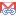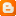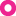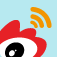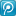### 3.4.4.Filter column position

In fact, this can not be considered separately, because there may be a number of filter columns, each filter column has its own location. We first consider the case of only one filter column.

select /*+full(a)*/a, b, c, d, e from T_PEEKING11 a where col < :v2;

TABROWS: 1000000; TABBLKS: 1000; QRYCOLNUMS: 5

 FLTCOLPOS COST_CPU CPU_B cpu_A 1 211121440 7121440 204000000 2 230121440 7121440 223000000 3 249121440 7121440 242000000 4 268121440 7121440 261000000

Well, change is linear, and in accordance with the assumption 4. Therefore, we need to derive the FLTCOLPOS in the formula of TYPFAC. In the formula 6, there exists two uncertain factors, so we have to solve a system of linear equations of four unknowns. Here we just modified one uncertain factor, and therefore can only come to two equations, it is not sufficient to solve:

(X1*1+Z1)*5 + (X2*1+Z2) = 204000000/1000000      … …      (1)

(X1*2+Z1)*5 + (X2*2+Z2) = 223000000/1000000      … …      (2)

Then we modify another factor, QRYCOLNUMS:

select /*+full(a)*/a, b, c, d from T_PEEKING11 a where col < :v2;

TABROWS: 1000000; TABBLKS: 1000; QRYCOLNUMS: 4

 FLTCOLPOS COST_CPU CPU_B cpu_A 1 210121440 7121440 203000000 2 229121440 7121440 222000000 3 248121440 7121440 241000000 4 267121440 7121440 260000000

We get the other 2 equations:

(X1*1+Z1)*4 + (X2*1+Z2) = 203000000/1000000      … …      (3)

(X1*2+Z1)*4 + (X2*2+Z2) = 222000000/1000000      … …      (4)

(2) – (1), (4) – (3) =>

X1*5 + X2 = 19

X1*4 + X2 = 19

=>

X1 = 0

=>

X2 = 19

Then solve the system of linear equations of two unknowns:

Z1*5 + 19 + Z2 = 204

Z1*4 + 19 + Z2 = 203

=>

Z1 = 1

Z2 = 180

We got the new formula of TYPFAC

Formula 7: TYPFAC = QRYCOLNUMS + 19*FLTCOLPOS + 180

### 3.4.5.Effective columns number

This concept maybe hard to understand. It comes from the special cases that mismatched the formula I ever got. Look at the following test data:

select /*+full(a)*/a from T_PEEKING11 a where c < :v2;

 COST_CPU CPU_B cpu_A 247121440 7121440 240000000

select /*+full(a)*/a, b from T_PEEKING11 a where c < :v2;

 COST_CPU CPU_B cpu_A 247121440 7121440 240000000

select /*+full(a)*/a, b, d from T_PEEKING11 a where c < :v2;

 COST_CPU CPU_B cpu_A 248121440 7121440 241000000

The first test data queried one column, and the second data queried two columns, but their results are the same! The third one queried three columns, but its result is not the same as the previous 2! Such a result is initially confused me. It seems that formula 4&6 are incorrect. And when I try these three data into the formula 7, found the results are incorrect:

1 +19 * 3 +180 = 238! = 240000000/1000000

2 +19 * 3 +180 = 239! = 240000000/1000000

3 +19 * 3 +180 = 240! = 241000000/1000000

OK! The process seems to have erred, or the one of our assumption is invalid. Let us carefully look at these special cases to see if we can find any rules.

Look at the first and second figures - the number of the query column has been changed, but it did not change COST_CPU. Here I have noticed one commonality between them: the location position of query columns are smaller than the filter column position (a : 1; b: 2; c: 3)! Whether can we draw such an assumption 5: the query columns that whose location position is less than or equal to the location position of the query filter columns will not be included in the effective columns. Many random testing data show that this assumption is valid, (can not find any anti-case, testing data will no longer be listed).

Then, where is the QEYCOLNUMS in formula 4 come from? Based on the formula 7, we can calculate the QEYCOLNUMS of first & second testing data:

240000000/1000000 - 180 - 19 * 3 = 3

3, what does this figure mean? I found the location position of the filter (FLTCOLPOS) is also 3, is a coincidence (or perhaps the finding is a coincidenceJ). Base on this finding, I set up assumption 6: when the query column is not effective column (or the location of query column is less than or equal to filter column), the number of query columns (we will call it effective column number) is the query column position (that is, the number of the query column and the column located before it). Similarly, I also adopted a number of random data to prove the assumption (can not find any anti-case).

However, these two assumptions are still not enough to explain a third data. In accordance with the formula 7, the QRYCOLNUMS = 241000000/1000000 - 180 - 19 * 3 = 4, does not equal to its column position (3). Don’t worry, according to the assumption 5, a, b two columns can not be calculated in the effective column, but the d is not in the scope of the assumption. If it is considered as an effective column, together with the assumption 6 (effective column from the filter column is 3), come to (3 +1) = 4. So we can draw an assumption 7: the columns whose location position is greater than the query filter will also be included in the effective columns. We can also prove the assumption by a number of random data.

OK, is it all of the impact of filter columns position? Let us now look at another group of testing data:

 select /*+full(a)*/X from T_PEEKING11 a where c < :v3; X COST_CPU CPU_B CPU_A d 512873940 7121440 505752500 e 512876440 7121440 505755000 f 512878940 7121440 505757500 g 512881440 7121440 505760000 d, e 512876440 7121440 505755000 d, f 512878940 7121440 505757500 f, g 512881440 7121440 505760000 d, g ,h 512883940 7121440 505762500

From the calculated EFFCOLNUMS in the set of data, we can easily find the rule: The max query column location position decides the number of effective query filed. This can also become one of our assumptions (8), at the same time, a groups of data can prove it.

From the above assumptions can be drawn:

Formula 8: EFFCOLNUMS = EFFQRYCOLNUM + FLTCOLPOS

EFFCOLNUMSeffective column number

FLTCOLPOSfilter column number

EFFQRYCOLNUMeffective query column. If there is any query column whose position greater than the filter column position, it will be the result of they subtraction max query column position and filter column postion, otherwise, it will be 0.

The QRYCOLNUMS in formula 7 has become EFFCOLNUMS,

Formula 9TYPFAC = EFFCOLNUMS + 19*FLTCOLPOS + 180

### 3.4.6.Max filter column position

Note: In case of multi-filter columns, we will first derived the case with only AND, and will finally involve the OR.

After complete of the previous derivation, I should deduced the impact of the number of filter column in the formula. However, the specific changes in the test data let me note that a factor under the conditions of multi-filter columns: the max filter column position.

Look at following set of data: two filter columns, with the same max filter column position: